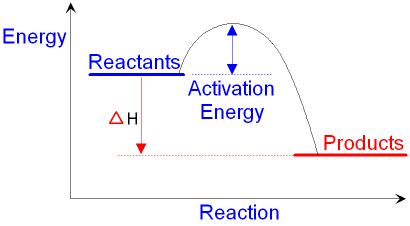gcsescience.com                                       26                                       gcsescience.com

Rates of Reaction

What are Energy Level Diagrams?

The change in energy of a chemical reaction can be plotted
against its progress as the reactants turn into products. The
energy level diagram for an exothermic reaction is shown below.

Going from the reactants to the top of the curve,
we are going up the energy axis of the graph.
Energy (heat) is being put in to break bonds in the reactants.

At the top of the curve, the bonds in the reactants
have been broken. The amount of energy put in
to break these bonds is called the activation energy.

The activation energy is the minimum amount of energy
needed for the reaction to occur. A catalyst can
work by lowering the activation energy for a reaction.Going from the top of the curve to the products,
we are going down the energy axis of the graph.
Energy (heat) is given out as bonds form in the products.

The reactants are higher up the energy axis
than the products. The amount of energy (heat)
you need to put in (the activation energy) is
less than the amount of energy (heat) you get out.
This is a typical exothermic reaction.

The difference in energy levels between
the reactants and the products
is given the symbol ΔH (pronounced 'delta H').
This is the amount of heat that is
given out or taken in during a
chemical reaction.

For an exothermic reaction, ΔH is negative.
For an endothermic reaction, ΔH is positive.

gcsescience.com        The Periodic Table        Index        Energy Quiz        gcsescience.com